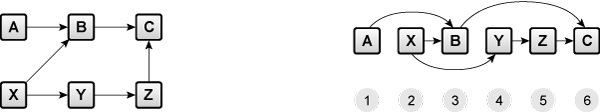# Path/Cycle - Topological Sort

Time Limit : 1 sec, Memory Limit : 131072 KB
Japanese version is here

# Topological SortA directed acyclic graph (DAG) can be used to represent the ordering of tasks. Tasks are represented by vertices and constraints where one task can begin before another, are represented by edges. For example, in the above example, you can undertake task B after both task A and task B are finished. You can obtain the proper sequence of all the tasks by a topological sort.

Given a DAG $G$, print the order of vertices after the topological sort.

## Input

A directed graph $G$ is given in the following format:
$|V|\;|E|$
$s_0 \; t_0$
$s_1 \; t_1$
:
$s_{|E|-1} \; t_{|E|-1}$

$|V|$ is the number of vertices and $|E|$ is the number of edges in the graph. The graph vertices are named with the numbers $0, 1,..., |V|-1$ respectively.

$s_i$ and $t_i$ represent source and target nodes of $i$-th edge (directed).

## Output

Print the vertices numbers in order. Print a number in a line.

If there are multiple possible solutions, print any one of them (the solution is judged by a special validator).

## Constraints

• $1 \leq |V| \leq 10,000$
• $0 \leq |E| \leq 100,000$
• There are no parallel edges in $G$
• There are no self loops in $G$

## Sample Input

6 6
0 1
1 2
3 1
3 4
4 5
5 2


## Sample Output

0
3
1
4
5
2